## 5.4.2 Simulated Annealing Algorithms

Simulated Annealing is a stochastic computational method for finding global extremums to large optimization problems. It was first proposed as an optimization technique by Kirkpatrick in 1983  and Cerny in 1984 . The optimization problem can be formulated as a pair of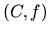, where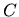describes a discrete set of configurations (i.e. parameter values) and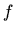is the objective function that is to be optimized. The problem is then to find a set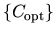such that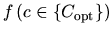is optimal.

The optimization algorithm is based on a Physical Annealing analogy. Physical Annealing is a process in which a solid is first heated until all particles are randomly arranged in a liquid state, followed by a slow cooling process. At each (cooling) temperature enough time is spent for the solid to reach thermal equilibrium, where energy levels follow Boltzmann distribution. As temperature decreases the probability tends to concentrate on low energy states. Care must be taken to reach thermal equilibrium prior to decreasing the temperature. At thermal equilibrium, the probability that a system is in a macroscopic configuration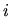with energy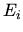is given by the Boltzmann distribution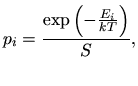(5.9)

with the set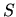of all possible configurations (or states)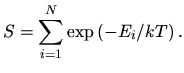(5.10)

Here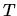is the absolute temperature,the Boltzmann constant,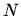the total number of configurations, and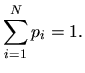(5.11)

The behavior of a system of particles can be simulated using a stochastic relaxation technique developed by Metropolis et al : Starting at time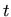and configuration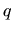a candidate configuration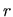for the time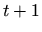is generated randomly. The new candidate is accepted or rejected based on the difference between the energies associated with statesand. The condition forto be accepted is determined by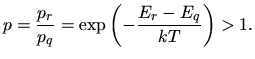(5.12)

If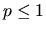thenis accepted with probability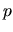. It was shown that for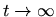, the probability that the system is in configuration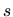equals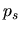. One feature of the Metropolis algorithm is that a transition out of a local minimum is always possible at nonzero temperature. Another evenly interesting property of the algorithm is that it performs a kind of adaptive divide and conquer: Gross features of the system appear at higher temperatures, fine features develop at lower temperatures.

Optimization by Simulated Annealing involves the following preparatory steps:

1. The analogies of the physical concept and the optimization problem must be determined. The energy function becomes a so-called cost function, the configuration of particles becomes the configuration of the parameters of the problem to optimize. Temperature is the control parameter of the optimization.

2. An annealing schedule needs to be selected that defines a decreasing set of temperatures and also the amount of time to spend at each temperature.

3. A way to generate and select new states must be defined.

The optimization algorithm is therefore comprised of three basic functional relationships: a probability density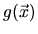, where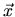is a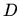-dimensional vector, the acceptance function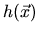, and the annealing schedule function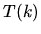with the time step. The optimization itself takes place iteratively. Initially, the algorithm starts from a randomly chosen point of which the cost is computed. Next a new point gets chosen from a random number generator with a probability density. In case the cost of this point is better than the cost of the other one, the new point is taken over. In case the cost is worse the point is accepted by a probability. Another point is always chosen based on the best point so far. With each iteration the probabilities for large deviations from the best point and for acceptance decrease. This results in a behavior where distant points are explored at the beginning (high temperature), but not generated or rejected respectively as the temperature cools down.

For the standard Boltzmann annealing,andare given by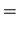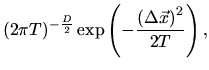(5.13)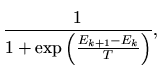(5.14)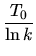(5.15)

with the deviation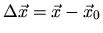of the new state from the previous one. Fig. 5.14 and Fig. 5.15 depict a plot of the probability densityand of the acceptance functionrespectively for a one dimensional optimization problem.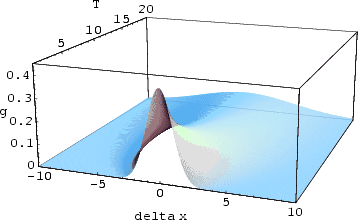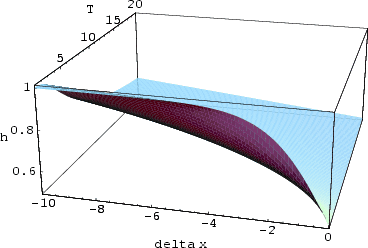Several different annealing schemes than the one defined in the original algorithm have evolved in the past. A fixed schedule which is characterized by a fixed initial temperature, a constant temperature decrement, and a constant number of steps at each temperature is usually not practically applicable to general optimization problems since it requires several tests with different parameter values. Adaptive algorithms, which change their parameters during the evolution of the cost function are more appropriate. An adaptive algorithm was presented by Huang . Here the parameters are chosen according to the mean value and standard deviation of the cost function. Also, in case the evaluation of the cost function itself is computationally expensive, a parallelized version of the Simulated Annealing algorithm is preferred over a sequential one.

Subsections

2003-03-27